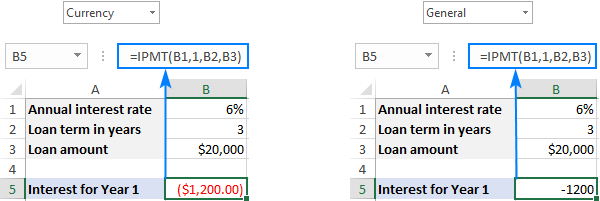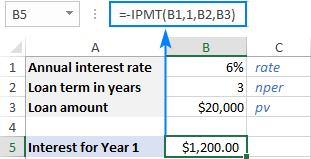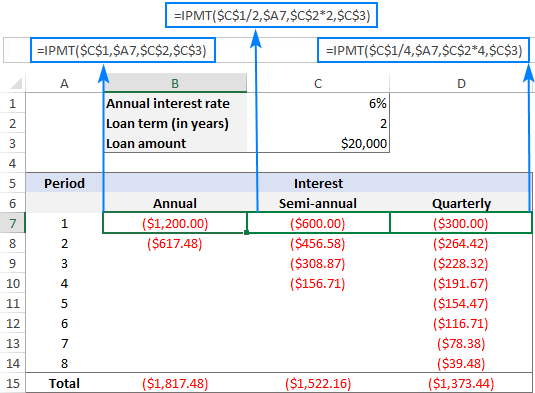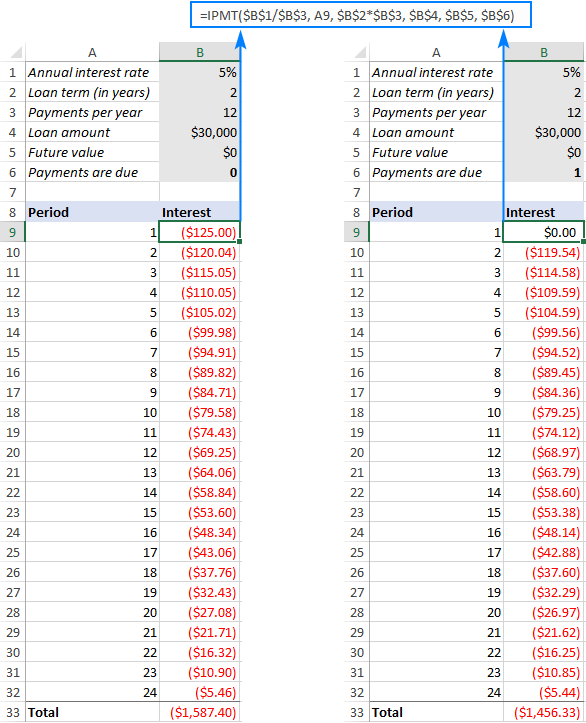# Excel IPMT function to calculate interest portion of a loan payment

The tutorial shows how to use the IPMT function in Excel to find the interest portion of a periodic payment on a loan or mortgage.

Whenever you take out a loan, whether it's a mortgage, home loan or car loan, you need to pay back the amount you originally borrowed and interest on top of it. In simple terms, interest is the cost of using someone's (usually a bank's) money.

The interest portion of a loan payment can be calculated manually by multiplying the period's interest rate by the remaining balance. But Microsoft Excel has a special function for this - the IPMT function. In this tutorial, we will go in-depth explaining its syntax and providing real-life formula examples.

## Excel IPMT function - syntax and basic uses

IPMT is Excel's interest payment function. It returns the interest amount of a loan payment in a given period, assuming the interest rate and the total amount of a payment are constant in all periods.

To better remember the function's name, notice that "I" stands for "interest" and "PMT" for "payment".

The syntax of the IPMT function in Excel is as follows:

IPMT(rate, per, nper, pv, [fv], [type])

Where:

• Rate (required) - the constant interest rate per period. You can supply it as a percentage or decimal number.

For example, if you make annual payments on a loan with an annual interest rate of 6 percent, use 6% or 0.06 for rate.

If you make weekly, monthly, or quarterly payments, divide the annual rate by the number of payment periods per year, as shown in this example. Say, if you make quarterly payments on a loan with an annual interest rate of 6 percent, use 6%/4 for rate.

• Per (required) - the period for which you want to calculate the interest. It must be an integer in the range from 1 to nper.
• Nper (required) - the total number of payments during the lifetime of the loan.
• Pv (required) - the present value of the loan or investment. In other words, it is the loan principal, i.e. the amount you borrowed.
• Fv (optional) - the future value, i.e. the desired balance after the last payment is made. If omitted, it is implied to be zero (0).
• Type (optional) - specifies when the payments are due:
• 0 or omitted - payments are made at the end of each period.
• 1 - payments are made at the beginning of each period.

For example, if you received a loan of \$20,000, which you must pay off in annual installments during the next 3 years with an annual interest rate of 6%, the interest portion of the 1st year payment can be calculated with this formula:

`=IPMT(6%, 1, 3, 20000)`

Instead of supplying the numbers directly into a formula, you can input them in some predefined cells and refer to those cells like shown in the screenshot below.

In accordance with the cash flow sign convention, the result is returned as a negative number because you pay out this money. By default, it is highlighted in red and enclosed in parenthesis (Currency format for negative numbers) as shown in the left part of the screenshot below. On the right, you can see the result of the same formula in the General format.If you'd rather get interest as a positive number, put a minus sign before either the entire IPMT function or the pv argument:

`=-IPMT(6%, 1, 3, 20000)`

or

`=IPMT(6%, 1, 3, -20000)`## Examples of using IPMT formula in Excel

Now that you know the basics, let's see how to use the IPMT function to find the amount of interest for different frequencies of payment, and how changing the loan conditions changes the potential interest.

Before we dive in, it should be noted that IPMT formulas are best to be used after the PMT function that calculates the total amount of a periodic payment (interest + principal).

### IPMT formula for different payment frequencies (weeks, months, quarters)

To get the interest portion of a loan payment right, you should always convert the annual interest rate to the corresponding period's rate and the number of years to the total number of payment periods:

• For the rate argument, divide the annual interest rate by the number of payments per year, assuming the latter is equal to the number of compounding periods per year.
• For the nper argument, multiply the number of years by the number of payments per year.

The following table shows the calculations:

 Frequency of payment Rate argument Nper argument Weekly annual interest rate / 52 years * 52 Monthly annual interest rate / 12 years * 12 Quarterly annual interest rate / 4 years * 4 Semi-annual annual interest rate / 2 years * 2

As an example, let's find the amount of interest you will have to pay on the same loan but in different payment frequencies:

• Annual interest rate: 6%
• Loan duration: 2 years
• Loan amount: \$20,000
• Period: 1

The balance after the last payment is to be \$0 (the fv argument omitted), and the payments are due at the end of each period (the type argument omitted).

Weekly:

`=IPMT(6%/52, 1, 2*52, 20000)`

Monthly:

`=IPMT(6%/12, 1, 2*12, 20000)`

Quarterly:

`=IPMT(6%/4, 1, 2*4, 20000)`

Semi-annual:

`=IPMT(6%/2, 1, 2*2, 20000)`

Looking at the screenshot below, you can notice that the interest amount decreases with each subsequent period. This is because any payment contributes to reducing the loan principal, and this reduces the remaining balance on which interest is calculated.

Also, please notice that the total amount of interest payable on the same loan differs for annual, semi-annual and quarterly installments:### Full form of the IPMT function

In this example, we are going to calculate interest for the same loan, the same payment frequency, but different annuity types (regular and annuity-due). For this, we will need to use the full form of the IPMT function.

To begin with, let's define the input cells:

• B1 - annual interest rate
• B2 - loan term in years
• B3 - number of payments per year
• B4 - loan amount (pv)
• B5 - future value (fv)
• B6 - when the payments are due (type):
• 0 - at the end of a period (regular annuity)
• 1 - at the beginning of a period (annuity due)

Assuming the first period number is in A9, our interest formula goes as follows:

`=IPMT(\$B\$1/\$B\$3, A9, \$B\$2*\$B\$3, \$B\$4, \$B\$5, \$B\$6)`

Note. If you plan to use the IPMT formula for more than one period, please mind the cell references. All the references to the input cells shall be absolute (with the dollar sign) so they are locked to those cells. The per argument must be a relative cell reference (without the dollar sign like A9) because it should change based on the relative position of a row to which the formula is copied.

So, we enter the above formula in B9, drag it down for the remaining periods, and get the following result. If you compare the numbers in the Interest columns (regular annuity on the left and annuity-due on the right), you will notice that interest is a little lower when you pay at the beginning of period.## Excel IPMT function not working

If your IPMT formula throws an error, it is most likely to be one of the following:

1. #NUM! error occurs is the per argument is out of the range 1 to nper.
2. #VALUE! error occurs if any of the arguments is non-numeric.

That's how you use the IPMT function in Excel. To have a closer look at the formulas discussed in this tutorial, you are welcome to download our Excel IPMT function sample workbook. I thank you for reading and hope to see you on our blog next week!

### 2 responses to "Excel IPMT function to calculate interest portion of a loan payment"

1.Francis says:

Hi Svetlana:
I have a insurance premium calculation done on excel and would like to embed it in my website so people can check the prices for life insurance. Could you please advise.
Thanks.
Francis